# 3D plot – Part 2

### Modeling Surfaces, Meshes and 3D variations

The 3D plot functions intended for plotting meshes and surfaces 'mesh' and 'surf', and their several variants 'meshc', 'meshz', 'surfc', and 'surfl', take multiple optional input arguments, the most simple form being 'mesh(z)' or 'surf(z)', where z represents a matrix.

 Usually, tridimensional curves are represented by the values of z-coordinates samples on a grid of (x,y) values. Thus, to create a surface or 3D plot we first need to generate a grid of (x,y) coordinates and find the height (z-coordinate) of the surface at each of the grid points. Matlab provides the function 'meshgrid' to create a grid of points over a specified range.

Meshgrid
Suppose that you want to plot the function z = x2 – 10y + 2 over the domain 0 ≤ x ≤ 4 and 0 ≤ y ≤ 4. To do so, we first take several points in the domain, say 25 points, as shown in this Fig.: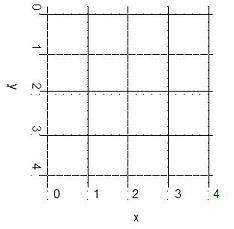We can create two matrices x and y, each of size 5 x 5, and write the xy-coordinates of each point in these matrices. We can then evaluate z with the command z = x.^2 – 10*y + 2.

However, creating the two matrices x and y is much easier with the meshgrid command.

% creates vectors x and y, from 0 to 4
vx = 0 : 4
vy = vx
% creates meshgrid to be used in 3D plot
[x,y] = meshgrid(vx,vy)

The commands shown above generate the 25 points shown in the figure. All we need to do is generate two vectors, vx and vy, to define the region of interest and distribution or density of our grid points. Also, the two vectors need not be either same sized or linearly spaced. It is very important to understand the use of meshgrid.

Matlab response is as follows:

vx =
0     1     2     3     4

vy =
0     1     2     3     4

x =
0     1     2     3     4
0     1     2     3     4
0     1     2     3     4
0     1     2     3     4
0     1     2     3     4

y =
0     0     0     0     0
1     1     1     1     1
2     2     2     2     2
3     3     3     3     3
4     4     4     4     4

 See the columns of x and the rows of y. When a surface is plotted with the 'mesh(z)' command (where z is a matrix), the tickmarks on the x and y axes do not indicate the domain of z but the row and column indices of the z-matrix. Typing 'mesh(x,y,z)' or 'surf(x,y,z)' (where x and y are vectors used by 'meshgrid' to create a grid), result in the surface plot of z, with x and y values shown along the respective axes.

The folowing script could be an example of how tho use the 'meshgrid', 'plot3', 'meshc', and 'surfc' commands.

We'll 3D plot the following surface: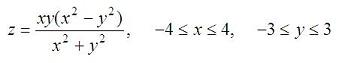with this script:

% clears command window, clears variables and closes figures
clc; clear; close all

% defines vectors x and y
vx = -4 : 0.2: 4;
vy = -3 : 0.2: 3;

% calculates the necessary grid
[x,y] = meshgrid(vx, vy);

% calculates z and avoids a null denominator adding 'eps'
% (eps is the least possible number in Matlab)
z = x .* y .* (x.^2 - y.^2) ./ (x.^2 + y.^2 + eps);

% generates the first figure using 'plot3'
figure
plot3(x,y,z)
grid on

% generates the second figure using 'meshc' to include the
% contour in the figure, and rotates the figure with 'view'
figure
meshc(x,y,z)
view(-37, 15)

% generates the third 3D figure using 'surfc' to include the
% contour in the image, and also rotates the figure with 'view'
figure
surfc(x,y,z)
view(-47, 25)

And the generated results are: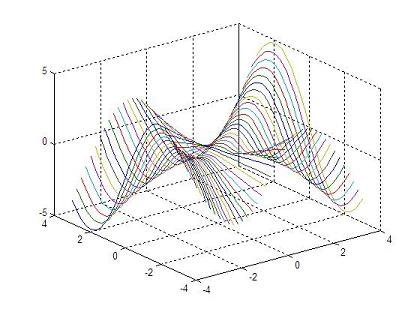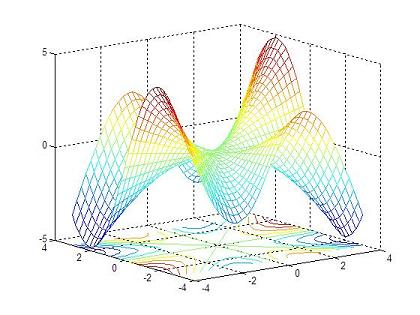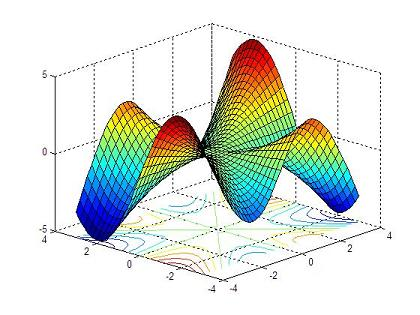Do you like it?

Use the instruction 'rotate3d on' to manipulate the view angle of your plot. Include it in your script or type it in the command window to change the view with your mouse over the figure...

From '3D Plot part 2' to home

From '3D Plot part 2' to 3D Main